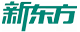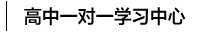#400-817-1616

## 计算老出错？小学数学简便算法归类，不能错过！

来源：网络

时间： 2019-07-15 16:01

小学数学中，从一年级到六年级一直贯穿着一个内容，那就是简便运算。

在整数范围、小数范围、分数范围内都作为一个内容重复出现，而这个内容也正是小学数学中的一个难点。

提取公因式

这个方法实际上是运用了乘法分配律，将相同因数提取出来，考试中往往剩下的项相加减，会出现一个整数，要注意相同因数的提取。

例：

0.92×1.41＋0.92×8.59

=0.92×（1.41+8.59）

借来还去法

看到名字，就知道这个方法的含义。用此方法时，需要注意观察，发现规律。还要注意，有借有还，再借不难。

考试中，看到有类似998、999或者1.98等接近一个非常好计算的整数的时候，往往使用借来借去法。

例：

9999+999+99+9

=9999+1+999+1+99+1+9+1-4

拆分法

顾名思义，拆分法就是为了方便计算把一个数拆成几个数。这需要掌握一些“好朋友”，如：2和5，4和5，2和2.5，4和2.5，8和1.25等。分拆还要注意不要改变数的大小哦。

例：

3.2×12.5×25

=8×0.4×12.5×25

=8×12.5×0.4×25

加法结合律

注意对加法结合律(a＋b)＋c=a＋(b＋c)的运用，通过改变加数的位置来获得更简便的运算。

例：

5.76＋13.67＋4.24＋6.33

=（5.76＋4.24）＋(13.67＋6.33)

乘法结合律

这种方法要灵活掌握拆分法和乘法分配律，在考卷上看到99、101、9.8等接近一个整数的时候，要首先考虑拆分。

例：

34×9.9=34×(10－0.1)

利用基准数

在一系列数中找出一个比较折中的数字来代表这一系列的数字，当然要记得这个数字的选取不能偏离这一系列数字太远。

例：

2072+2052+2062+2042+2083

=（2062x5）+10-10-20+21

利用公式法

(1)加法：

交换律，a+b=b+a

结合律，(a+b)+c=a+(b+c)

(2)减法运算性质：

a-(b+c)=a-b-c

a-(b-c)=a-b+c

a-b-c=a-c-b

(a+b)-c=a-c+b=b-c+a

(3)乘法（与加法类似）：

交换律，a*b=b*a，

结合律，（a*b）*c=a*(b*c)，

分配率，（a+b）*c=ac+bc

(a-b)*c=ac-bc

(4)除法运算性质（与减法类似）：

a÷(b*c)=a÷b÷c

a÷（b÷c)=a÷bxc

a÷b÷c=a÷c÷b

(a+b)÷c=a÷c+b÷c

(a-b)÷c=a÷c-b÷c

前边的运算定律、性质公式很多是由于去掉或加上括号而发生变化的。其规律是同级运算中，加号或乘号后面加上或去掉括号，后面数值的运算符号不变。

例1：

283+52+117+148

=（283+117）+（52+48）

（运用加法交换律和结合律）

减号或除号后面加上或去掉括号，后面数值的运算符号要改变。

例2：

657-263-257

=657-257-263

=400-263

（运用减法性质，相当加法交换律）

例3：

195-（95+24）

=195-95-24

=100-24

（运用减法性质）

例4：

150-(100-42)

=150-100+42

(同上)

例5：

（0.75+125）*8

=0.75*8+125*8

=6+1000

=1006

(运用乘法分配律)

例6：

（125-0.25）*8

=125*8-0.25*8

=1000-2

=998

(同上)

例7：

（1.125-0.75）÷0.25

=1.125÷0.25-0.75÷0.25

=4.5-3

=1.5

（运用除法性质）

例8：

(450+81)÷9

=450÷9+81÷9

=50+9

=59

(同上，相当乘法分配律)

例9：

375÷（125÷0.5）

=375÷125*0.5

=3*0.5

=1.5

(运用除法性质)

例10：

4.2÷（0.6*0.35）

=4.2÷0.6÷0.35

=7÷0.35

=20

(同上)

例11：

12*125*0.25*8

=(125*8)*(12*0.25)

=1000*3

=3000

(运用乘法交换律和结合律)

例12：

(175+45+55+27)-75

=175-75+(45+55)+27

=100+100+27

=227

(运用加法性质和结合律）

例13：

（48*25*3）÷8

=48÷8*25*3

=6*25*3

=450

(运用除法性质，相当加法性质)

裂项法

分数裂项是指将分数算式中的项进行拆分，使拆分后的项可前后抵消，这种拆项计算称为裂项法。

常见的裂项方法是将数字分拆成两个或多个数字单位的和或差。遇到裂项的计算题时，要仔细地观察每项的分子和分母，找出每项分子分母之间具有的相同的关系，找出共有部分，裂项的题目无需复杂的计算，一般都是中间部分消去的过程，这样的话，找到相邻两项的相似部分，让它们消去才是最根本的。

分数裂项的三大关键特征：

（1）分子全部相同，最简单形式为都是1的，复杂形式可为都是x(x为任意自然数)的，但是只要将x提取出来即可转化为分子都是1的运算。

（2）分母上均为几个自然数的乘积形式，并且满足相邻2个分母上的因数“首尾相接”。

（3）分母上几个因数间的差是一个定值。

（责任编辑：刘汉甜）